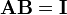# Inverse matrix

In mathematics, a square matrix A may have a left-inverse matrix A−1 defined by$\mathbf{A}^{-1}\mathbf{A} = \mathbf{I} := \begin{pmatrix} 1 & 0 & 0 & \cdots & 0 \\ 0 & 1 & 0 & \cdots & 0 \\ 0 & 0 & 1 & \cdots & 0 \\ \cdots & & & & \\ 0 & 0 & 0 & \cdots & 1 \\ \end{pmatrix}.$

If A−1 exists, the matrix A is called regular, non-singular, or invertible.

If for an invertible matrix A it holds that$\mathbf{A}\mathbf{B} = \mathbf{I}$

then the matrix B is the right-inverse of A. Assume that A is invertible and multiply the last equation by the left-inverse$\mathbf{A}^{-1}\mathbf{A}\mathbf{B} = \mathbf{A}^{-1}\mathbf{I} = \mathbf{A}^{-1} \quad\Longrightarrow\quad \mathbf{B}= \mathbf{A}^{-1}.$

It follows that for any finite-dimensional matrix A the right-inverse matrix is equal to the left-inverse matrix, simply called the inverse of A and indicated by A−1.

A necessary and sufficient condition for the inverse A−1 to exist is the non-vanishing of the determinant: det(A) ≠ 0.

See this article for an explicit expression for the elements of A−1.